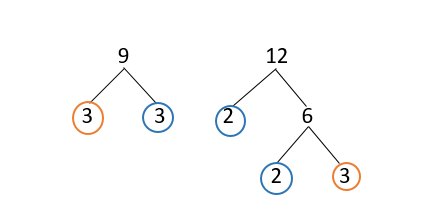# Help Zone

### Student Question

My teacher tells me that to work on fractions, it is useful to master LCM. How can I find it? I have heard of multiples and trees.

Mathematics{t c="richEditor.description.title"} {t c="richEditor.description.paragraphMenu"} {t c="richEditor.description.inlineMenu"} {t c="richEditor.description.embed"}

## Explanations (1)

•Explanation from AlloprofExplanation from Alloprof

This Explanation was submitted by a member of the Alloprof team.

Good evening,

The multiples method and the factor tree method are indeed used to find the LCM! There is a third technique, the table, but let's tackle the ones you mentioned!

1. Method of multiples.

To find the LCM, we first make a list of the multiples of each number.

Suppose the numbers 6, 9 and 12.

The multiples of 6 = {6, 12, 18, 24, 30, 36, 42, 48,…}

The multiples of 9 = {9, 18, 27, 36, 45, 54 , 63, 72,…}

The multiples of 12 = {12, 24, 36, 48, 60, 72, 84, 96,…}

We observe that 36 is the lowest common multiple!

2. Factor tree method.

Find the LCM of two numbers:

We decompose the two numbers using a factor tree until we get only prime factors. Common factors and unique factors are identified.

Example 9 and 12.We get 9 = 3 x 3.

We get 12 = 2 x 2 x 3.

The common factor (in orange) is 3.

The unique factors (in blue) are 3, 2 and 2.

The common factors are multiplied by the single factors.

So 3 x 3 x 2 x 2 = 36 which is the LCM.